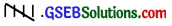# GSEB Class 7 Maths Notes Chapter 3 Data Handling

This GSEB Class 7 Maths Notes Chapter 3 Data Handling covers all the important topics and concepts as mentioned in the chapter.

## Data Handling Class 7 GSEB Notes

Today data handling is one of the most important tasks in any organisation. In hospitals data needs to be maintained. In schools cumulative records of students are kept for further reference.

Data handling includes collection, interpretation or presentation of data by using various methods.
1. Data: A data is a collection of facts in the form of numerical figures that is used to provide some information.

2. Observation: Each numerical figure (entry) in a data is called an observation (or variate)

3. Frequency: The number of times a particular observation occurs in the data is called its frequency.

4. Tally Marks: Tally marks are useful in counting observations. We write tally marks in bunches of five like5. Arrayed data: A data arranged in ascending or descending order is called arrayed data.

6. Frequency Table: A table showing the frequency of various observations is called a frequency distribution table or frequency table.

7. Arithmetic Mean: Arithmetic mean usually termed a mean, is one of the measures of central tendency as it gives the average value of the given data.

The average or Arithmetic Mean (A.M.) or simply mean is defined as follows:
Mean = $$\frac{\text { Sum of all observations }}{\text { Number of observations }}$$

8. Range: The difference between the highest observation and the lowest observation is called the range of the data. [Range = Highest observation – Lowest observation].

• Mode: The observation which occurs maximum number of times in the given data is called mode (or modal value) of the data.
• Mode of Larger Data: Putting the same observations together and counting them is not easy if the number of observations is large. In such cases we tabulate the data. Tabulation can begin by putting tally marks and finding the frequency.
• Median: Median is that value which lies in the middle of the data. When observations are arranged in ascending or descending order with half of the observations above it and the other half below it.

Bar Graphs
A bar graph is a representation of numbers using bars of uniform width. In bar graphs, the height (or length) of a bar represents the frequency of the corresponding observation. All bars must be of equal width and there should be equal gap between the adjoining bars.

Choosing a scale
In a bar graph where numbers in units are to be shown, the graph represents one unit length for our observation. If it has to show number in tens or hundreds one length can represent 10 or 100 observations.

Double Bar Graphs
A graph that displays two sets of data using two bars drawn besides each other is called a double bar graph. This graph helps us to compare two collections of data at a glance.Chance
Chance is the occurrence of events. It is the possibility of something happening.
Look at the statements given below and try to understand these terms.

• The sun rises from the west.
• An ant growing to 3 m height.
• India winning the next cricket match.

If we look at the statements given above we would say that the sun rises from the West is impossible, an ant growing to 3 m is also not possible. Where as India can win the match or lose it. Both are possible.

Probability
When a die is thrown probability of getting either of 1, 2, 3, 4, 5 or 6 is equal. For a die, there are 6 equally likely possible outcomes. We say that each of 1, 2, 3, 4, 5, 6 has one – sixth $$\frac{1}{6}$$ probability.

The probability of an event E, written as P (E), is defined as
P(E) = $$\frac{\text { Number of favourable outcomes }}{\text { Total number of outcomes }}$$
Number of favourable outcomes Total number of outcomes We shall study more about this in next classes.

Event that have many possibilities can have probability between 0 and 1. Those which have no chance of happening have probability 0 and those that are bound to happen have a probability 1.

• An experiment is a situation that involves a chance of the occurrence of a particular event.
• An outcome is the result of an experiment.
• Sample space is the set of all possible outcomes in an experiment.
• An event is a specific outcome of an experiment.